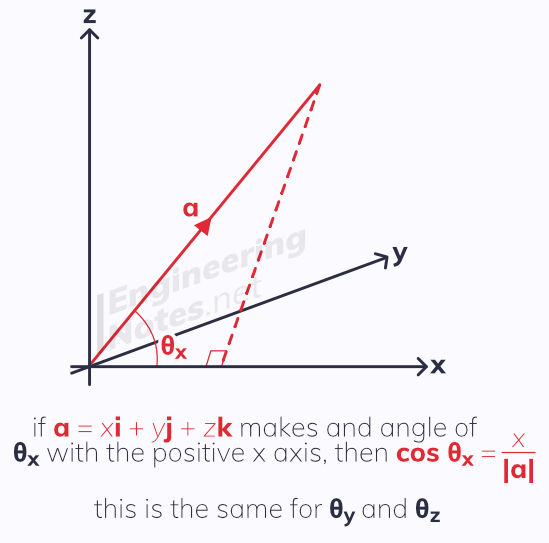top of page

#### Notes by Category University Engineering

Rate these notesNot a fanNot so goodGoodVery goodBrillRate these notes

# Vectors

A vector is a quantity with both magnitude and direction, and is typically represented visually by a line segment between two points.There are many ways of representing vectors in notation:

• The two points that the vector connects with an arrow above them

• As a bold typeface lower case letter

• As an underlined lower case letter

• As a column vector, showing displacement in the x-direction above that in the y-direction

• As a multiple of unit vectors, i (one unit in the positive x-direction) and j (one unit in the positive y-direction)

These notes will predominantly use bold typeface of lower case letters, underlined letters and the unit vectors i and j.Vectors can be multiplied by a scalar, and added and subtracted:## Magnitude & Direction

The magnitude of a vector is given by Pythagoras' Theorem. Magnitude is noted using straight lines on either side of the letter, like modulus.

For the vector a = xi + yj, |a| = √(x²+y²)

A unit direction vector, â, can be found as a / |a|

A vector can also be defined by giving its magnitude and the angle it makes with one of the coordinate axis. This is called magnitude-direction form.

## Position Vectors

Position vectors are used to give the location of a point relative to a fixed origin.A point (p, q) has a position vector pi + qj

### Vector Geometry

Position vectors can be used to solve geometric problems:## Vectors in 3D

In three-dimensional geometry, there are x, y, and z-axes. This means each coordinate has three values, (x, y, z). The position vector of this is xi + yj + zkPythagoras' Theorem still applies, but adjusted for three points:

Distance from point (x, y, z) to origin is given as √(x²+y²+z²)The distance between two points, (x₁, y₁, z₁) and (x₂, y₂, z₂) is given as:

√( (x₁ - x₂)² + (y₁ - y₂)² + (z₁ - z₂)² )

### Vector Geometry in 3DSee All
bottom of page